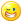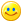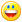## Welcome to the Second Life Forums Archive

These forums are CLOSED. Please visit the new forums HERE

# Multidimensional Arrays! (well... sort of)

Christopher Omega
Oxymoron
Join date: 28 Mar 2003
Posts: 1,828
12-18-2003 15:42
Reposted to the script library from the Scripts ans Scripting forum:

Hi everyone!

I'd like to share a few lines of code with the community, that a group of very good friends and I collaborated on.

Verbose version (w/ many comments):

CODE
`// Multidimensional Array API v0.1// Written by Christopher Omega, edited by... many people// Goal: To psuedo-impliment multidimensional array capability into LSL that allows the user to// use the API as if it were a regular, plain-old datatype of the language itself.// Acknowledgements:// I'd like to personally thank all who've helped/pitched-in during the development of this API, including:// Eggy Lippman// Mezzanine Peregrine// Malichi Petunia// Antagonistic Protagonist// Rhysling Greenacre// and last, but not least, Ama Omega.// NOTES ON THE API:// Instead of standard multidimensional array formatting which looks like [a][r],// the API uses the LSL list format for representing array indexes, the equivelant of the previous standard array index// would be [b,a,r].This method is used to eliminate parsing overhead in the script.// I do not believe that the API currently supports 'ragged' arrays, otherwise known as non-rectangular arrays,//____START Global Functions____// initArray() - Initilizes lists to be used with the array API.// Arguments:// arrayBounds == a list representing the bounds of the array to be created. (See notes for formatting.)list initArray(list arrayBounds) {    return [llList2CSV(arrayBounds)];}// NOTE: ALL FUNCTIONS ASSUME THAT ARRAY HAS BEEN INITILIZED USING initArray().// getArrayBounds() - Gets the maximum amount of elements you can put in each dimension, in list format.// Arguments:// array == the array to get the bounds of.list getArrayBounds(list array){    // Declaring array equal to its bounds is a bit confusing, I just do it to clear up the memory and    // do the operation at the same time.        array = llCSV2List(llList2String(array,0)); // List representing bounds of array.    return array;}// isValidBounds() - Tests to see if arrayIndex is valid within array.// Arguments:// arrayIndex == list representing the array index to check. (See notes for formatting.)// arrayBounds == list containing the bounds of the array arrayIndex is in. (get this val with getArrayBounds().)integer isValidBounds(list arrayBounds, list arrayIndex){    // reqDims defined here to decrease cycles used when 'for' loop iterates.    // reqDims stores the number of dimensions arrayIndex referrs to.    integer reqDims = llGetListLength(arrayIndex);        integer arrayDims = llGetListLength(arrayBounds);    if(reqDims != arrayDims)    {        return FALSE; //Cant refer to more dimensions then the array has.    }    else    {        integer i;        for(i = 0; i < reqDims; i++) // Loop over every dimension.         {            integer bounds = (integer)llList2String(arrayBounds,i); //Bounds of dimension in array.            integer index = (integer)llList2String(arrayIndex,i); //Value of the dimension of the arrayIndex you want to check.            if(index < 0){return FALSE;}            if(index >= bounds){return FALSE;}        }        return TRUE;    }}        // getFlatIndexOf() - Returns 'flat' (1-d) index of the specific arrayIndex.// Arguments:// arrayIndex == specific list representing the array index you want to convert. (See notes for formatting.)// arrayBounds == list containing the bounds of the array arrayIndex is in. (get this val with getArrayBounds(),)integer getFlatIndexOf(list arrayBounds, list arrayIndex){        // Equation for an array with max indices [X][Y][Z][A] when you want element [x][y][z][a] is    // index = x * (Y * Z * A) + y * (Z * A) + z * (A) + a. (Note the capatalization).    // Ty to Ama Omega for the above formula.    if(isValidBounds(arrayBounds,arrayIndex))    {        integer reqDimNum = llGetListLength(arrayIndex); // Number of dimensions arrayIndex represents.        integer arrayDimNum = llGetListLength(arrayBounds); // Number of dimensions in array.                // Thanks a *ton* Mezz for the code snippet below.                //[Start Mezzanine]        // okay, we have dimensions of the array        // Note: an 4 dimensional array only has to declare 3 of its dimensions.        // since the 4'th is not used.                // so you could feed a BOGUS number in as the first dimension and it wouldnt care                // I just kept it there to keep it simple.                // however, for this example, I will assume that        // All four dimensions are in the list        // like such : [A,B,C,D,E]        // meaning a C or java style array        // of [A][C][D][E]        //: so first coordinate multiplied by all except the first dimension PLUS second coordinate multiplied by all except the first two dimensions PLUS third coord multiplied by all except the first thee dimensions            integer coord_iter;        integer dim_iter;        integer accumulator;        integer multiplicant;                accumulator = 0;                for (coord_iter = 0; coord_iter < arrayDimNum; coord_iter++)        {            // load the current coordinate.            multiplicant = llList2Integer(arrayIndex,coord_iter);            // now we loop thru the dimensions starting AFTER the current coordinate            // and ending at the last coord.            if (coord_iter != arrayDimNum - 1)            {                for (dim_iter = coord_iter + 1;dim_iter < arrayDimNum; dim_iter++)                {                    multiplicant = multiplicant * llList2Integer(arrayBounds,dim_iter);                }            }            // add the offset            accumulator += multiplicant;        }        return accumulator + 1;         //[End Mezz]        //About return- First element of list is metadata, so add 1 to result to offset.    }    return -1;}// getData() - returns string value of element in array, at arrayIndex.// Arguments:// arrayIndex == specific list representing the array index of the element in the array.// array == the array that arrayIndex is inlist getData(list array, list arrayIndex)  {    list bounds = getArrayBounds(array);        integer indexLen = llGetListLength(arrayIndex);    integer boundsLen = llGetListLength(bounds);        if(indexLen < boundsLen) //Wants list of elements.    {        if(indexLen == 0) // Simply return what was given to the function.        {            return array;        }        else        {            list minArrayIndex = arrayIndex; // The minimum array index, the first value you want to get.            list maxArrayIndex = arrayIndex; // The max. array index, the last val you want to get.            integer i;            for(i = indexLen; i < boundsLen; i++) // Iterate until every dimension is filled in.            {                integer maxBound = (integer)llList2String(bounds,i) - 1;                maxArrayIndex += maxBound;                minArrayIndex += 0;            }                        return llList2List(array,getFlatIndexOf(bounds,minArrayIndex),getFlatIndexOf(bounds,maxArrayIndex));            // The above return works because every value is sequential in the 1-d list,  resides above ,            // if the list represents an array with bounds .        }                    }    else //Wants only one element.    {        integer trueIndex = getFlatIndexOf(bounds,arrayIndex);        return llList2List(array,trueIndex,trueIndex);    }}//____Start setData() functions____// changeDataAt() - returns list with newData inserted into src at index, newData will always be at index,// therefore, sometimes indices need to be filled with spacers ("".// Arguments:// src == list to impliment the change into. (Keep in mind, LSL is pass by value.)// index == index in src to put newData.// newData == a list of data to insert into src at index. (Currently only can be one element.)list changeDataAt(list src, integer index, list newData){    integer dataLen = llGetListLength(newData);    integer i;    for(i = 0; i < index + dataLen; i++)    {        if(llGetListEntryType(src,i) == TYPE_INVALID) //If there's no data in the index.        {            src = llListInsertList(src,[""],i); //Fill the index with a spacer.        }    }    src = llDeleteSubList(src,index,index + dataLen - 1);    src = llListInsertList(src,newData,index);    return src;}// setData() - Sets the element at arrayIndex in array, to data.// Arguments:// arrayIndex == specific list representing the array index you want to get data from.// array == the array that arrayIndex is in.// data == the new data.list setData(list array, list arrayIndex, list data){    integer trueIndex = getFlatIndexOf(getArrayBounds(array),arrayIndex);    return changeDataAt(array,trueIndex,data);}//End setData functions...//___End global functions___`

Non-verbose, more optimized version w/o comments:

CODE
`// Multidimensional Array API v0.1// Written by Christopher Omega, edited by... many people// Goal: To psuedo-impliment multidimensional array capability into LSL that allows the user to// use the API as if it were a regular, plain-old datatype of the language itself.// Acknowledgements:// I'd like to personally thank all who've helped/pitched-in during the development of this API, including:// Eggy Lippman// Mezzanine Peregrine// Malichi Petunia// Antagonistic Protagonist// Rhysling Greenacre// and last, but not least, Ama Omega.// NOTES ON THE API:// Instead of standard multidimensional array formatting which looks like [a][r],// the API uses the LSL list format for representing array indexes, the equivelant of the previous standard array index// would be [b,a,r].This method is used to eliminate parsing overhead in the script.// I do not believe that the API currently supports 'ragged' arrays, otherwise known as non-rectangular arrays.//____START Global Functions____list initArray(list arrayBounds) {    return [llList2CSV(arrayBounds)];}list getArrayBounds(list array){    return llCSV2List(llList2String(array,0));}integer isValidBounds(list arrayBounds, list arrayIndex){    integer reqDims = llGetListLength(arrayIndex);        integer arrayDims = llGetListLength(arrayBounds);    if(reqDims != arrayDims){return FALSE;}    else    {        integer i;        for(i = 0; i < reqDims; i++)        {            integer bounds = (integer)llList2String(arrayBounds,i);            integer index = (integer)llList2String(arrayIndex,i);                        if(index < 0){return FALSE;}            if(index >= bounds){return FALSE;}        }        return TRUE;    }}integer getFlatIndexOf(list arrayBounds, list arrayIndex){    if(isValidBounds(arrayBounds,arrayIndex))    {        integer reqDimNum = llGetListLength(arrayIndex);        integer arrayDimNum = llGetListLength(arrayBounds);                // [Start Mezz]        // okay, we have dimensions of the array        // Note: an 4 dimensional array only has to declare 3 of its dimensions.        // since the 4'th is not used.                // so you could feed a BOGUS number in as the first dimension and it wouldnt care                // I just kept it there to keep it simple.                // however, for this example, I will assume that        // All four dimensions are in the list        // like such : [A,B,C,D,E]        // meaning a C or java style array        // of [A][C][D][E]        //: so first coordinate multiplied by all except the first dimension PLUS second coordinate multiplied by all except the first two dimensions PLUS third coord multiplied by all except the first thee dimensions            integer coord_iter;        integer dim_iter;        integer accumulator;        integer multiplicant;                accumulator = 0;                for (coord_iter = 0; coord_iter < arrayDimNum; coord_iter++)        {            // load the current coordinate.            multiplicant = llList2Integer(arrayIndex,coord_iter);            // now we loop thru the dimensions starting AFTER the current coordinate            // and ending at the last coord.            if (coord_iter != arrayDimNum - 1)            {                for (dim_iter = coord_iter + 1;dim_iter < arrayDimNum; dim_iter++)                {                    multiplicant = multiplicant * llList2Integer(arrayBounds,dim_iter);                }            }            // add the offset            accumulator += multiplicant;        }        return accumulator + 1;         //[End Mezz]    }    return -1;}list getData(list array, list arrayIndex)  {    list bounds = getArrayBounds(array);        integer indexLen = llGetListLength(arrayIndex);    integer boundsLen = llGetListLength(bounds);    if(indexLen < boundsLen)    {        if(indexLen == 0){return array;}        else        {            list maxArrayIndex = arrayIndex;            integer i;            for(i = indexLen; i < boundsLen; i++)            {                integer maxBound = (integer)llList2String(bounds,i) - 1;                maxArrayIndex += maxBound;                arrayIndex += 0;            }                        return llList2List(array,getFlatIndexOf(bounds,arrayIndex),getFlatIndexOf(bounds,maxArrayIndex));        }                    }    else    {        integer trueIndex = getFlatIndexOf(bounds,arrayIndex);        return llList2List(array,trueIndex,trueIndex);    }}list changeDataAt(list src, integer index, list newData){    integer dataLen = llGetListLength(newData);    integer i;    for(i = 0; i < index + dataLen; i++)    {        if(llGetListEntryType(src,i) == TYPE_INVALID)        {            src = llListInsertList(src,[""],i);        }    }    src = llDeleteSubList(src,index,index + dataLen - 1);    return llListInsertList(src,newData,index);}list setData(list array, list arrayIndex, list data){    return changeDataAt(array,getFlatIndexOf(getArrayBounds(array),arrayIndex),data);}//____End Global Functions____`

Example usage:

CODE
`default{    state_entry()    {                llSay(0,"Warning, spam ahead, starting loop.");        llSay(0,"Creating array...");        list foo;        foo = initArray([2,2,2,2]);        integer i;        integer j;        integer k;        integer l;        for(i = 0; i < 2; i++)        {            for(j = 0; j < 2; j++)            {                for(k = 0; k < 2; k++)                {                    for(l = 0; l < 2; l++)                    {                        string str = "[" + (string)i + "][" + (string)j + "][" + (string)k + "][" + (string)l + "]";                        foo = setData(foo,[i,j,k,l],[str]);                    }                }            }        }        llSay(0,"Done generating array.");                llSay(0,llList2CSV(getData(foo,[0,0])));    }}`

This is basicly a moderately sophisticated way of using a strided list. Credit for the original concept goes to Eggy-----------------------------
Tips and tricks:
The API supports getting lists of data.

Basicly, you pass an array index with a lesser number of dimensions then the number of dimensions the array contains.

What it does is convert your index into two full indexes, one with the lowest index you want, and one with the highest.

So, if you pass it [a][c], when the array has bounds [A][B[C][D], you get the list from [a][c] to [a][c][D - 1].

When you pass it something with even lesser bounds, say [a] you get the list from [a] to [a][C - 1][D - 1].
-----------------------------

[Licensing]
This API is completely free to use. I humbly ask that the acknowledgments and author name of this API exist in scripts that impliment it.
To make this even more clear, you don't need to put in the acknowledgments/author, I'd just appreciate it.
[/Licensing]

Whew! :wipes brow, cracks wrists: That coloring was *hard* to do by hand! Seems to make a difference in readability though, someone should make an auto-forum-code-colorer thingy!==Chrischechel Choche
Registered User
Join date: 1 May 2007
Posts: 5
01-25-2008 06:38
how can anybody use a script lsl and htmlcode mix ???

sorry but this here is mischmasch...

greetings CC